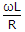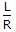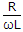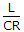# Electronics and Communication Engineering - Electromagnetic Field Theory

31.
Short-circuited stubs are preferred to open circuited stubs because the latter are
more difficult to make and connect
incapable of giving a full range of reactances
made of transmission line with a different characteristic impedance
Explanation:
No answer description is available. Let's discuss.

32.
The induction field of short current element varies as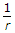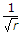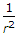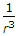Explanation:
No answer description is available. Let's discuss.

33.
In binomial arrays the elimination of secondary lobes takes place
at the cost of directivity
at the cost of gain
both (a) and (b)
none of the above
Explanation:
No answer description is available. Let's discuss.

34.
The characteristic equation of plane wave in E independent of two dimensions y and z is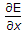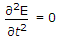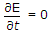none of the above